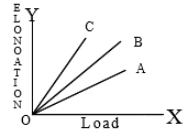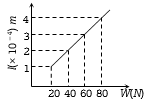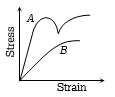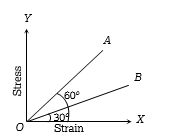You’ve reached the end of your free Videos limit.
#11 | Application of Elastic Behaviour of Material
(Physics) > Mechanical Properties of Solids
Unable to watch the video, please try another server
Related Practice Questions :

Three wires A,B,C made of the same material and radius have different lengths. The graphs in the figure show the elongation-load variation. The longest wire is1. A

2. B

3. C

4. All

High Yielding Test Series + Question Bank - NEET 2020

Difficulty Level:

The adjacent graph shows the extension $\left(∆l\right)$ of a wire of length 1m suspended from the top of a roof at one end with a load W connected to the other end. If the cross sectional area of the wire is ${10}^{-6}{m}^{2}$ calculate the young’s modulus of the material of the wire(a) $2×{10}^{11}N/{m}^{2}$

(b) $2×{10}^{-11}N/{m}^{2}$

(c) $3×{10}^{-12}N/{m}^{2}$

(d) $2×{10}^{-13}N/{m}^{2}$

High Yielding Test Series + Question Bank - NEET 2020

Difficulty Level:

The diagram shows stress v/s strain curve for the materials A and B. From the curves we infer that(a) A is brittle but B is ductile

(b) A is ductile and B is brittle

(c) Both A and B are ductile

(d) Both A and B are brittle

High Yielding Test Series + Question Bank - NEET 2020

Difficulty Level:

The stress versus strain graphs for wires of two materials A and B are as shown in the figure. If ${Y}_{A}$ and ${Y}_{B}$ are the Young ‘s modulii of the materials, then(a) ${Y}_{B}=2{Y}_{A}$

(b) ${Y}_{A}={Y}_{B}$

(c) ${Y}_{B}=3{Y}_{A}$

(d) ${Y}_{A}=3{Y}_{B}$